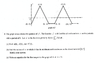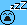# Minimum and Maximum Values

a1234

## Homework Statement

The problem is in the attached file. The part I need a little help with is part b.

## Homework Equations

and attempt at a solution[/B]

For part a, I got h(8) = 2, h'(6) = -2, and h''(4) = -2.
For part c, I found that the integral from 0 to 5 is 7, so I multiplied 7 by 7 to get 49 (because 35 = 5*7). And f(5) = 0, so f(108) also equals 0. I got the tangent line y - 49 = 0(x - 35).

For part b, I think we're supposed to consider x = 0 and x = 3, since this is where the graph starts increasing from decreasing and vice versa. But I also think we have to evaluate the integral at these points. Can someone explain this?

#### Attachments

•Calculus.PNG
25.7 KB · Views: 400

Homework Helper
Gold Member
2022 Award
found that the integral from 0 to 5 is 7
The period is not 5, so why is that interesting?

Homework Helper
If I consider
I think we're supposed to consider x = 0 and x = 3
as an attempt, I agree with 0 and 3 but I don't see it decrease before 0... I also see another interval where f is not bigger than at 0 ...

If you want help for part (a) -- and I think you need it -- , please post your working in detail.

 strike because the question is about ##h## and I mistakenly read ##f## --- bedtimeHomework Helper
Gold Member
2022 Award
If you want help for part (a) -- and I think you need it
Did you make a similar mistake here? I agree with all the answers for a).

•BvU
Homework Helper
YES ! aaaarrghh

a1234
The period is not 5, so why is that interesting?

I thought the graph would keep repeating every 5 units, but that's not true.
It seems to repeat every 8 units, so could we do this?

integral from 0 to 8 = 1
8*4 = 32
1*4 = 4
integral from 0 to 3 = 3
4 + 3 = 7
y - 7 = 0(x - 35)?

Homework Helper
Gold Member
2022 Award
It seems to repeat every 8 units
You are told it does.
integral from 0 to 8 = 1
Didn"t you calculate it as 2 in part a?
y - 7 = 0(x - 35)?
I do not understand that step. What is the gradient of h at x=35?

a1234
I didn't count the triangle from 0 to 1 when I first calculated the integral from 0 to 8. I think it should be 1 because the area of the trapezoid is -6, area of the large triangle is 8, and area of the small triangle is -1. So 8 + (-6) + (-1) = 1.

For the derivative at x = 35...
h(35) = integral from 0 to 35 = 7
h'(35) = f(35) = 4 because the graph repeats every 8 units...f(3) = 4 for the remaining 3 units after 8*4 = 32. So the derivative at x = 35 is 4.
If I put it into point-slope form, y - 7 = 4(x - 35) is the tangent line.

Homework Helper
Gold Member
2022 Award
the area of the trapezoid is -6
The trapezoid extends to x=9.

a1234
Ugh. I'm making a lot of mistakes here.

You're right, we only need the area of the trapezoid up to x = 8. So then the area of the trapezoid is 5, and h(8) = 2. The integral from 0 to 35 is 2*4 + 3 = 11.
y - 11 = 4(x - 35)

Homework Helper
Gold Member
2022 Award
Ugh. I'm making a lot of mistakes here.

You're right, we only need the area of the trapezoid up to x = 8. So then the area of the trapezoid is 5, and h(8) = 2. The integral from 0 to 35 is 2*4 + 3 = 11.
y - 11 = 4(x - 35)
Looks right.

a1234
How do I go about part b?

Homework Helper
Gold Member
2022 Award
How do I go about part b?
Do you understand about local extrema and absolute extrema?
How do you find a local extremum?

a1234
The absolute maximum is the highest point over the entire graph. The absolute minimum is the lowest point over the graph. The relative max/min is the maximum or minimum over a certain interval.

I think the first step is to find do h'(x), which is just f(x) and find the critical points and endpoints of the graph. The critical points are 1 and 5, and the endpoints are 0 and 7. And then we need to evaluate g at those values. I came up with the following:
h(0) = 0
h(1) = -1
h(5) = 7
h(7) = 4
The absolute min is -1 and max is 7. The relative min is 0 and max is 4.